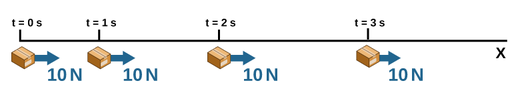Acceleration is the rate at which velocity changes over time. Since velocity is a vector, it may change in magnitude, in direction, or both; and thus acceleration must also be a vector quantity. The vector of acceleration is in the same direction as the change in velocity.

As expressed by Newton's Second Law of motion, the acceleration of a system is directly proportional to and in the same direction as the net external force acting on the system and is inversely proportional to its mass.

An example of acceleration produced by a force is the acceleration produced by Earth’s gravity. Since gravity is directly proportional to the mass of the object it accelerates and acceleration produced by a force on an object is inversely proportional to the mass of the object, the dependencies on mass cancel out and the acceleration produced by Earth’s gravity is the same for objects of any mass. Near the surface of Earth, this acceleration is approximately 9.8 m/s^2 .Figure 1: Box accelerating as the result of the external force.